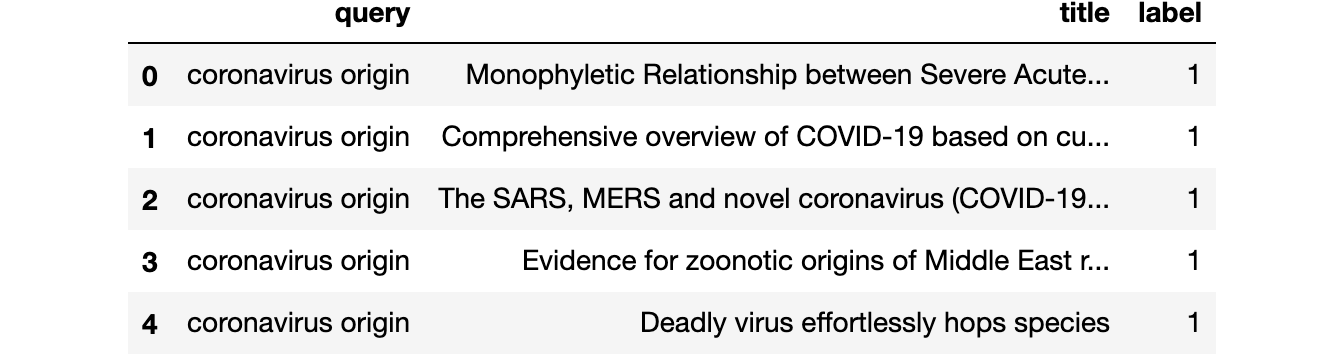Thiago Martins
Vespa Data Scientist

# Fine-tuning a BERT model with transformers

Setup a custom Dataset, fine-tune BERT with Transformers Trainer and export the model via ONNX.

This post describes a simple way to get started with fine-tuning transformer models. It will cover the basics and introduce you to the amazing `Trainer` class from the `transformers` library. I will leave important topics such as hyperparameter tuning, cross-validation and more detailed model validation to followup posts.Photo by Samule Sun on Unsplash

We use a dataset built from COVID-19 Open Research Dataset Challenge. This work is one small piece of a larger project that is to build the cord19 search app.

You can run the code from Google Colab but do not forget to enable GPU support.

## Install required libraries

``````pip install pandas transformers
``````

In order to fine-tune the BERT models for the cord19 application we need to generate a set of query-document features as well as labels that indicate which documents are relevant for the specific queries. For this exercise we will use the `query` string to represent the query and the `title` string to represent the documents.

``````training_data = read_csv("https://thigm85.github.io/data/cord19/cord19-query-title-label.csv")
``````There are 50 unique queries.

``````len(training_data["query"].unique())
50
``````

For each query we have a list of documents, divided between relevant (`label=1`) and irrelevant (`label=0`).

``````training_data[["title", "label"]].groupby("label").count()
``````## Data split

We are going to use a simple data split into train and validation sets for illustration purposes. Even though we have more than 50 thousand data points when we consider unique query and document pairs, I believe this specific case would benefit from cross-validation since it has only 50 queries containing relevance judgement.

``````from sklearn.model_selection import train_test_split
train_queries, val_queries, train_docs, val_docs, train_labels, val_labels = train_test_split(
training_data["query"].tolist(),
training_data["title"].tolist(),
training_data["label"].tolist(),
test_size=.2
)
``````

## Create BERT encodings

Create train and validation encodings. In order to do that we need to chose which BERT model to use. We will use padding and truncation because the training routine expects all tensors within a batch to have the same dimensions.

``````from transformers import BertTokenizerFast

tokenizer = BertTokenizerFast.from_pretrained(model_name)

train_encodings = tokenizer(train_queries, train_docs, truncation=True, padding='max_length', max_length=128)
val_encodings = tokenizer(val_queries, val_docs, truncation=True, padding='max_length', max_length=128)
``````

## Create a custom dataset

Now that we have the encodings and the labels we can create a `Dataset` object as described in the transformers webpage about custom datasets.

``````import torch

class Cord19Dataset(torch.utils.data.Dataset):
def __init__(self, encodings, labels):
self.encodings = encodings
self.labels = labels

def __getitem__(self, idx):
item = {key: torch.tensor(val[idx]) for key, val in self.encodings.items()}
item['labels'] = torch.tensor(self.labels[idx])
return item

def __len__(self):
return len(self.labels)

train_dataset = Cord19Dataset(train_encodings, train_labels)
val_dataset = Cord19Dataset(val_encodings, val_labels)
``````

### Fine-tune the BERT model

We are going to use `BertForSequenceClassification`, since we are trying to classify query and document pairs into two distinct classes (non-relevant, relevant).

``````from transformers import BertForSequenceClassification

model = BertForSequenceClassification.from_pretrained(model_name)
``````

We can set `requires_grad` to `False` for all the base model parameters in order to fine-tune only the task-specific parameters.

``````for param in model.base_model.parameters():
``````

We can then fine-tune the model with `Trainer`. Below is a basic routine with out-of-the-box set of parameters. Care should be taken when chosing the parameters below, but this is out of the scope of this piece.

``````from transformers import Trainer, TrainingArguments

training_args = TrainingArguments(
output_dir='./results',          # output directory
evaluation_strategy="epoch",     # Evaluation is done at the end of each epoch.
num_train_epochs=3,              # total number of training epochs
per_device_train_batch_size=16,  # batch size per device during training
per_device_eval_batch_size=64,   # batch size for evaluation
warmup_steps=500,                # number of warmup steps for learning rate scheduler
weight_decay=0.01,               # strength of weight decay
save_total_limit=1,              # limit the total amount of checkpoints. Deletes the older checkpoints.
)

trainer = Trainer(
model=model,                         # the instantiated 🤗 Transformers model to be trained
args=training_args,                  # training arguments, defined above
train_dataset=train_dataset,         # training dataset
eval_dataset=val_dataset             # evaluation dataset
)

trainer.train()
``````

### Export the model to onnx

Once training is complete we can export the model using the ONNX format to be deployed elsewhere. I assume below that you have access to a GPU, which you can get from Google Colab for example.

``````from torch.onnx import export

device = torch.device("cuda")

model_onnx_path = "model.onnx"
dummy_input = (
train_dataset["input_ids"].unsqueeze(0).to(device),
train_dataset["token_type_ids"].unsqueeze(0).to(device),